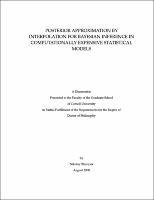## Posterior Approximation by Interpolation for Bayesian Inference in Computationally Expensive Statistical Models##### Abstract
Markov Chain Monte Carlo (MCMC) is nowadays a standard approach to numerical computation of integrals of the posterior density $\pi$ of the parameter vector $\eta$. Unfortunately, Bayesian inference using MCMC is computationally intractable when $\pi$ is expensive to evaluate. In this work, we develop practical methods that approximate $\pi$ with radial basis functions (RBFs) and Gaussian processes (GPs) interpolants and use the resulting cheap-to-evaluate surfaces in MCMC. In Chapter 1, $\pi$ arises from a nonlinear regression model with transformation and dependence. To build the RBF approximation, we limit evaluation of the computationally expensive regression function to points chosen on a high posterior density (HPD) region found using a local quadratic approximation of $\log(\pi)$ at its mode. We illustrate our approach on simulated data for a pollutant diffusion problem and study the frequentist coverage properties of credible intervals. In Chapter 2, we relax the assumptions about $\pi$ made in Chapter 1 and develop a derivative-free procedure GRIMA to approximate the logarithm of $\pi$ using RBF interpolation over a HPD region of $\pi$ estimated using the RBF surface. We use GRIMA for Bayesian inference in a computationally intensive nonlinear regression model for real measured streamflow data in the Town Brook watershed. In Chapter 3, we study statistical models where it is possible to identify a minimal subvector $\beta$ of $\eta$ responsible for the expensive computation in the evaluation of $\pi$. We propose two approaches to approximate $\pi$ by interpolation that exploit this computational structure. Our primary contribution is derivation of a GP interpolant that provably improves over some of the existing approaches by reducing the effective dimension of the interpolation problem from $\dim(\eta)$ to $\dim(\beta)$. When $\dim(\eta)$ is high but $\dim(\beta)$ is low, this allows one to dramatically reduce the number of expensive evaluations necessary to construct an accurate approximation of $\pi$. Our experiments indicate that our methods produce results similar to those when the true expensive posterior density is sampled by MCMC while reducing computational costs by well over an order of magnitude.